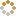﻿ Ningbo University of Technology Online Judge ::  Final number
• ###  Final number

• 时间限制: 1000 ms　内存限制: 65535 K
• 问题描述
• There's a game called final number. It's like that:

The judger gives out a number and let no one knows. And then he will give out a range.

The players guess the number in the range one by one. The judge will update the range every time. So the range will be smaller and smaller until one player guesses out the number.

• 输入
• The input data include several cases, input until EOF.
In each case, The first line include 3 integers:
L, R (1 <= L < R <= 2147483647, means the range) and N (L < N < R, means the final number)
Then it will follow one integer T(1 <= T <= 1000, means the time the players guess) in a single line.
Then it follows T lines, each line include a integer, means the number one player guesses.
You may suppose all the numbers they guess are valid (That is, the numbers are in the range which is last updated) and the last one is the final number.
• 输出
• For each case, you will output the initial range, and for each time they guess, you should output the updated range in a single line. If the number is the final number, you should output 'Bingo!' in a single line.
• 样例输入
• ```1 100 75
6
20
90
45
60
80
75
```
• 样例输出
• ```1 - 100
20 - 100
20 - 90
45 - 90
60 - 90
60 - 80
Bingo!
```
• 提示
• `无`
• 来源
• `XadillaX`
• 操作
﻿